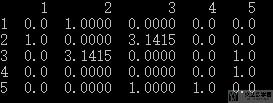# Python中图结构的操作-NetworkX介绍

• A+

## 图的一些操作

### 创建一个图

1. import networkx as nx
2. G = nx.Graph()

### 添加节点与添加边

2.     (4, {"color": "red"}),
3.     (5, {"color": "green"}),
4. ])

1. G.add_edges_from([(2, 3, {'weight': 3.1415}), (4, 5), (3, 5)])

### 顶点和边的信息

1. numNodes = G.number_of_nodes()
2. numEdges = G.number_of_edges()

1. list(G.nodes)
2. """
3. [1, 2, 3, 4, 5]
4. """
5. list(G.edges)
6. """
7. [(1, 2), (2, 3), (3, 5), (4, 5)]
8. """

### 判断node与node之间是否有边

1. nx.is_path(G, (2,3))
2. # >> True
3. nx.is_path(G, (2,4))
4. # >> False

## 图的可视化

1. import matplotlib.pyplot as plt
2. options = {
3.     'with_labels': True,
4.     'font_weight': 'bold',
5.     'node_color': 'blue',
6.     'node_size': 300,
7.     'width': 3,
8. }
9. nx.draw(G, **options)
10. plt.show()## 读取和保存文件

1. a b c # source target target
2. d e

1. #@dataPre.py
2. #@ GMT Thu Sep 10 18:41:01 2020
3. #@
4. 1 2
5. 2 3
6. 3 5
7. 4 5
8. 5

2.     """写入文件
3.     """
5.     numNodes = subG.number_of_nodes()
6.     numEdges = subG.number_of_edges()
7.     # 开始写入
8.     fh.write(('{} {}\n'.format(numNodes, numEdges)).encode('utf-8'))
9.     for line in nx.generate_adjlist(subG, " "):
10.         line += "\n"
11.         fh.write(line.encode('utf-8'))

1. 5 4
2. 1 2
3. 2 3
4. 3 5
5. 4 5
6. 5

## 转换为其他数据类型

### 转换为pandas的格式• 微信公众号
• 关注微信公众号
•• QQ群
• 我们的QQ群号
•